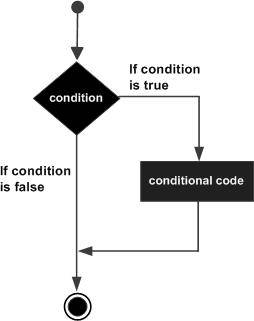## C++ if 语句C++ 判断

## 语法

C++ 中 if 语句的语法：

```if(boolean_expression)
{
// 如果布尔表达式为真将执行的语句
}
```

C 语言把任何非零非空的值假定为 true，把null 假定为 false

## 流程图## 实例

```#include <iostream>
using namespace std;

int main ()
{
// 局部变量声明
int a = 10;

// 使用 if 语句检查布尔条件
if( a < 20 )
{
// 如果条件为真，则输出下面的语句
cout << "a 小于 20" << endl;
}
cout << "a 的值是 " << a << endl;

return 0;
}
```

```a 小于 20
a 的值是 10
```C++ 判断